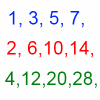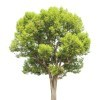# Patterns and Sequences - Stage 3### Days and Dates

##### Age 11 to 14 Challenge Level:

Investigate how you can work out what day of the week your birthday will be on next year, and the year after...### Summing Consecutive Numbers

##### Age 11 to 14 Challenge Level:

15 = 7 + 8 and 10 = 1 + 2 + 3 + 4. Can you say which numbers can be expressed as the sum of two or more consecutive integers?### 1 Step 2 Step

##### Age 11 to 14 Challenge Level:

Liam's house has a staircase with 12 steps. He can go down the steps one at a time or two at time. In how many different ways can Liam go down the 12 steps?### Frogs

##### Age 11 to 14 Challenge Level:

How many moves does it take to swap over some red and blue frogs? Do you have a method?### Picturing Triangular Numbers

##### Age 11 to 14 Challenge Level:

Triangular numbers can be represented by a triangular array of squares. What do you notice about the sum of identical triangle numbers?### Picturing Square Numbers

##### Age 11 to 14 Challenge Level:

Square numbers can be represented as the sum of consecutive odd numbers. What is the sum of 1 + 3 + ..... + 149 + 151 + 153?### Coordinate Patterns

##### Age 11 to 14 Challenge Level:

Charlie and Alison have been drawing patterns on coordinate grids. Can you picture where the patterns lead?### Squares in Rectangles

##### Age 11 to 14 Challenge Level:

A 2 by 3 rectangle contains 8 squares and a 3 by 4 rectangle contains 20 squares. What size rectangle(s) contain(s) exactly 100 squares? Can you find them all?### Triangle Numbers

##### Age 11 to 14 Challenge Level:

Take a look at the multiplication square. The first eleven triangle numbers have been identified. Can you see a pattern? Does the pattern continue?### Elevenses

##### Age 11 to 14 Challenge Level:

How many pairs of numbers can you find that add up to a multiple of 11? Do you notice anything interesting about your results?### Tower of Hanoi

##### Age 11 to 14 Challenge Level:

The Tower of Hanoi is an ancient mathematical challenge. Working on the building blocks may help you to explain the patterns you notice.### Shifting Times Tables

##### Age 11 to 14 Challenge Level:

Can you find a way to identify times tables after they have been shifted up or down?### Charlie's Delightful Machine

##### Age 11 to 16 Challenge Level:

Here is a machine with four coloured lights. Can you develop a strategy to work out the rules controlling each light?### What Numbers Can We Make?

##### Age 11 to 14 Challenge Level:

Imagine we have four bags containing a large number of 1s, 4s, 7s and 10s. What numbers can we make?### Odds, Evens and More Evens

##### Age 11 to 14 Challenge Level:

Alison, Bernard and Charlie have been exploring sequences of odd and even numbers, which raise some intriguing questions...### Seven Squares

##### Age 11 to 14 Challenge Level:

Watch these videos to see how Phoebe, Alice and Luke chose to draw 7 squares. How would they draw 100?### What Numbers Can We Make Now?

##### Age 11 to 14 Challenge Level:

Imagine we have four bags containing numbers from a sequence. What numbers can we make now?### Beach Huts

##### Age 11 to 14 Challenge Level:

Can you figure out how sequences of beach huts are generated?### Growing Surprises

##### Age 11 to 14 Challenge Level:

Can you find the connections between linear and quadratic patterns?### Square Grid

##### Age 11 to 14 Short Challenge Level:

Can you work out what fraction of this grid is shaded?### Tiled Floor

##### Age 11 to 14 Short Challenge Level:

This tiled floor has 109 purple tiles. How many tiles are there altogether?